Reach Us+44 7482 874137
The Development of a Label-Free Electrochemical Impedance Based Pointof-care Technology for Multimarker Detection | OMICS International
Journal of Biosensors & Bioelectronics

Like us on:

All submissions of the EM system will be redirected to Online Manuscript Submission System. Authors are requested to submit articles directly to Online Manuscript Submission System of respective journal.

# The Development of a Label-Free Electrochemical Impedance Based Pointof-care Technology for Multimarker Detection

Ugur Korcan Demirok1, Aman Verma2 and Jeffrey T La Belle1,2*

1The Bio design Institute at Arizona State University, Tempe, AZ, 85287, USA

2Harrington Program of Biomedical Engineering in the School of Health and Systems Engineering at Arizona State University, Tempe, AZ, 85287, USA

*Corresponding Author:
Jeffrey T La Belle
Harrington Program of Biomedical
Engineering in the School of Health
and Systems Engineering at Arizona State University
Tempe, AZ, 85287, postal: 550 East Orange Street
Tempe, AZ, 85287-9709, USA
Tel: 14807279061
Fax: 14807277624
E-mail: [email protected]

Received Date: April 24, 2013; Accepted Date: May 08, 2013; Published Date: May 10, 2013

Citation: Demirok UK, Verma A, La Belle JT (2013) The Development of a Label-Free Electrochemical Impedance Based Point-of-care Technology for Multimarker Detection. J Biosens Bioelectron S12:004. doi: 10.4172/2155-6210.S12-004

Copyright: © 2013 Demirok UK, et al. This is an open-access article distributed under the terms of the Creative Commons Attribution License, which permits unrestricted use, distribution, and reproduction in any medium, provided the original author and source are credited.

Visit for more related articles at Journal of Biosensors & Bioelectronics

#### Abstract

With an increasing global population, rising healthcare costs, and greater demand on hospitals and clinicians, a growing need for low cost, rapid, Point of Care Technologies (POCT) exists. The overall goal is to detect or monitor a disease in order to give patients and clinicians fast, accurate, and all-encompassing information regarding the state of the disease. A challenge for many currentpoint-of-care technologies is the difficulty of monitoring several biomarkers simultaneously without the complexity of multiple sensors, labels, or spatially separated transducers. We have previously shown that convoluted signals obtained from protein biomarkers monitored by electrochemical impedance spectroscopy can be “tuned” away from one another by conjugation with gold nanoparticles to allow for the potential simultaneous detection of multiple biomarkers. This method of detection yields a sensitive and specific means of biomarker quantification in human media including tears or blood. In this work, we detail the development of a mathematical model that explores the roles of various factors, such as nanoparticle size and the nature of materials, so that a design space could be created for tuningotherwise convoluted biomarkers. Furthermore, we present assessments as to the validity of this model with preliminary bench-top experiments by taking advantage of gold nanoparticle-antibody conjugates of varying sizes. Gold nanoparticle size changes of 5, 10, and 20 nm demonstrated a 10.0, 4.8, and 1.0 Hz shift in frequency, respectively. Future work includes exploration of different sensor configurations, continuous monitoring, and the prospect for implantable sensors were also discussed as potential future avenues.

#### Keywords

Nanoparticles; Electrochemical Impedance Spectroscopy; Tuning; Biosensors; Biomarkers

#### Introduction

Clinically, many diseases are assessed by the use of a single biomarker. Diabetes, for instance, is monitored daily via glucose concentrations, and prostate specific antigen (PSA) is commonly used to screen for prostate cancer . However, a single biomarker is oftennot sufficient for reliable disease monitoring, screening, or diagnosis. As a result, physicians routinely perform “blood work” to access the levels of several biomarkers in order to accurately access a patient’s condition . In a recent paper, our group demonstrated that Electrochemical Impedance Spectroscopy (EIS) can be used to conjugate gold nanoparticles to antibodies against IL-12, an interleukin produced physiologically in response to antigen stimulation . EIS is a powerful measurement technique for biosensing applications because of its unique advantages, including the ability to provide label-free detection, high sensitivity, faster assay, and short detection response times . EIS measures the resistance and capacitive properties of an electrochemical system upon perturbation of an AC signal. The AC signal has a varying potential that, upon contact with the system or material of interest, results in a corresponding current response. The frequency of the inputted AC signal with varying potential is swept over a wide range of frequencies to enable the calculation of complex impedance. The complex impedance consists of real and imaginary components, corresponding to resistance and reactance, respectively. Mathematically, the complex impedance is equated using the following relation: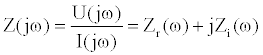(1)

Where, Z(jω) is the complex impedance, a function of the angular frequency ω , which is equal to 2πf; U(jω) is the applied potential; I(jω) is the current response; Zr is the real impedance; and jZi(ω) is the imaginary impedance.

Recent reports have indicated that the binding of different targetmolecular recognition pairs results in unique frequencies of optimal detection. This frequency of optimal binding is the frequency, under a concentration gradient of target that causes the most significant change in impedance as measured by responsivity and R-square. The basis of the frequency effect is due to many factors, spacing of charge, protein composition, the sensor material, linkers used in immobilization, among others. For instance, a detection frequency of 10 Hz was observed in Salmonella typhimurium, and multiple cytokine-antibody pairswere found to have unique and concentration-independent binding frequencies [5,6]. Nelson’s group in Leed’s demonstrated that with single amino acid substitutions, the EIS Nyquist spectra are drastically altered . However, an inherent challenge is that the frequency spectra are tightly limited for (typically 1 kHz on the 100,000 to 1.0 Hz spectra), and the Q factor or full-width half maxima are not especially tight for biological species.In the case of diabetes, we have determined glucose/ glucose oxidase interactions to occur at 1.17 kHz, and have also found that 1,5-anhydroglucitol, glycated albumin, glycated hemoglobin and insulininteract with their molecular recognition elements at 3.71 kHz, 1.465 kHz, 117 Hz, and 4.59 kHz, respectively . Due to the risk for overlap among biomarkers of similar frequency responses, methods for separation of maximal interaction frequencies of biomarkers are needed (Figure 1).

Figure 1: Schematic representation of maximum binding-interaction of target and molecular recognition elements for multiple diabetes markers. INSETclose up showing marker and actual frequency data of maximal interaction from experiments showing issue of potential overlap (NOTE frequency values are actual data but curves are NOT actual data but representation of shape of an idealized frequency response).

Since it was previously determined that the formation of nanoparticle-antibody conjugates is capable of “tuning” the response frequency of IL-12 antibodies (Figure 2) while maintaining antibody specificity , a mathematical model was developed herein to further explore the tuning possibilities of different nanoparticle-antibody conjugates based on selected nanoparticle materials and sizes. The motivation for constructing this model is the potential tobuild upon preliminary bench-top validation for the development of a design space for nanoparticle-conjugates, which canbe used in the eventualconstruction of a multiplexed biosensor using EIS.

Figure 2: (A) Conjugation of AuNP (a) with monoclonal antibody (b) forms AuNP Conjugated Mab complex. (B) The addition of AuNP changes frequency response of Mab from a lower frequency to a higher frequency.

#### Materials and Methods

Gold nanoparticle preparation and conjugation

The gold nanoparticles used in this study were prepared according to a method that has been described in the literature . All chemical and reagents used, Sigma-Aldrich (MO, USA) unless otherwise noted. Antibodies and antigens in lypholized form were purchased from R&D Systems, Minnesota, MN, and were reconstituted in phosphate buffered saline (PBS), purchased in tablet form from CalBioChem, La Jolla, CA, and was dissolved in DI water to yield a working solution of 140 mM NaCl, 10 mM phosphate buffer and 3 mM KCl, with a pH 7.4 at 25°C.

The conjugation of the antibodies with gold nanoparticles was performedaccording to the following procedures: The minimum amount of antibody required to stabilize the gold nanoparticles in a given solution was determined by mixing a series of concentrations of the protein (10-100 ug/mL, 1 mL) with a milliliter of gold solution and incubation for 5 min. Next, 0.5 mL of 10% (w/v) NaCl was added and the color of the solution observed. The concentration, just above that of the solution in which the gold changes color from red to blue was deemed as the minimum required amount for stabilization. Up to 25% excess protein was used above the minimum concentration level to ensure a high degree of stabilization.

The incubation of gold nanoparticles with the appropriate amount of antibody was conducted for 20 min at room temperature after bringing the pH of the colloidal suspension to pH 8-9 with K2CO3–close to the isoelectronic point of the immunoglobulin G (IgG) molecules. The resulting solution was then transferred to an appropriately-sized Beckman Quick-Seal tube and centrifuged in a Beckman-Coulter Optima L-100 XP Ultracentrifuge at various forces (50,000 g for 20 nm, 70,000 g for 10 nm, 90,000 g for 5 nm and 100,000 g for 2 nm) at 4°C for 1 hr to separate un conjugated antibodies from the gold-antibody complexes. A dark red-colored pellet obtained from centrifugation was then reconstituted in 10 mM phosphate buffered saline. The conjugates were used immediately, although reports exist in the literature indicating the stability of the antibody-gold complexes containing sodium azide (NaN3) at 4°C for months  suggesting the technique would have sufficient shelf-life.

Electrochemical sensor fabrication and validation of tuning range

Gold disc electrodes (2 mm diameter) purchased from CH Instruments, Inc., Austin, TX, were used throughout this study in order to assess the tuning capability of the gold nanoparticle-antibody conjugates. Gold electrodes were polished for 2 min with 1, 0.3, and 0.05 µm Al2O3 powder, and were sonicated for 5 min in acetone, ethanol, and DI water to remove alumina from the surface. Upon washing the electrodes with copious amounts of DI water, cyclic voltammetry was performed on the electrodes using a three-membered apparatus (Au working electrode, Pt counter electrode, and Ag/AgCl reference electrode) in a solution of 5 mM [Fe(CN)6]3-/[Fe(CN)6]4-, 0.1 M KCl, and 10 mM PBS (pH=7.4) to obtain the formal potential and a peak-to-peak separation of ~60 mV (59 mV theoretical). Impedance measurements were taken subsequently from an electrode at the former potential obtained from CVs at a frequency range of 0.1 Hz to 105 Hz, with amplitude of 5 mV using a CHI660C analyzer (CH Instruments, Inc.).

A self-assembling layer of 16-mercaptohexadecanoic (16-MHDA) acid was formed on the previously cleaned gold electrode through thiol linkages. The electrodes were kept in MHDA solutions witha concentration of 1 mM to 25 mM (in absolute ethanol) for a period varying between 1 to 12 hrs at room temperature. Most electrodes were immersed in 1 mM MHDA for 1 hr at RT since this setting was found to have a dense surface coverage while minimizing the background signal prior to further functionalization. Carboxylate groups of 16-MHDA were converted to succinimidyl esters by immersing the electrode in an aqueous solution of 40 mM 1-ethyl-3-(3-dimethylaminopropyl) carbodiimide (EDC) in the presence of 10 mM water-soluble sulfoderivative of N-hydroxysuccinimide (NHS) for 1 hr. After decanting the EDC-NHS and washing the sensor with DI water, the antibody (anti-IL-12 or anti-TNF-α) was then immobilized on the activated sensor surface by immersion into a 10 µg/mL solution (in PBS) of the desired antibody overnight at 4°C. The sensor was washed with PBS following immobilization and blocked by ethanolamine using an aqueous solution of 1% (w/v) for 30 min against non-specific binding, prior to antigen exposure at varying concentrations.

#### Mathematical Modeling

All mathematical modeling was conducted using MATLAB R2011b software (MathWorks, MA, USA)

The tuning of the frequency response from the impedance of the nanoparticle-antibody conjugates is based on the physics of electrical circuits (Figure 3). The frequency response of the nanoparticle alone, due to its resistive and capacitive properties from the complex impedance (Table 1), is added to the characteristic frequency response of the antibody in order to provide a change in frequency.

Figure 3: (A) exaggerated gold disk electrode-sensor showing (a) gold nanoparticle attached to (b) antibody, immobilized via self-assembled monolayer of (c) alkanethiol linker attached to (d) gold disk electrode (not to scale), (B) physical components of the sensor and their equivalent circuit model representations (determined experimentally), and (c) resulting equivalent circuit model of the electrode system.

Component Impedance
R ZR = R
C ZC = 1/jωC
W (Diffusion) ZW =1/Y0(jω) -1/2
Q (CPE) ZQ =1/Y1 (jω)α

Table 1: Equivalent circuit model components and resulting impedance.

The frequency is related to the resistance and capacitance according to the following equation: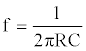(2)

Where, R is the resistance and C is the capacitance. Because nanoparticles are geometrical objects, the resistance formula selected for calculating the resistance R is that of a long, cylindrical wire of length L, cross-sectional area A, and resistivity ρ. As such, the appropriate resistance equation is the following: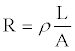(3)

The resistivity term ρ can be replaced in the above equation using the electrical conductivity of the nanoparticle σ, which can be determined using a number of different approaches [11,12]. A limitation of the above resistance formula is that the equation assumes the geometric object as a cylindrical rod, which means the equation has to be slightly modified to account for the spherical geometry of nanoparticles used in this study. To make the necessary modifications, the length term is converted to the circumference of a circle based on the radius r using the following equation:

L = 2π r (4)

The cross-sectional area term Aremains the same and can be calculated using the standard equation for the area of a circle. Therefore, the modified resistance formula for calculating the resistance of spherical nanoparticles of different radii is the following: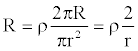(5)

The equation above exhibits asymptotic behavior when the radius is equal to zero. For this reason, a value of 0.1 nm is selected as the lowest radius for the spherical nanoparticles in the constructed mathematical model. Chaki et al.  formulated an equation for the capacitance of nanoparticle clusters based on the dielectric constant, radius, and spacing of the nanoparticles. Based on their models, the capacitance of a nanoparticle can be calculated using the following equation: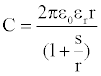(6)

Where ε0 is the dielectric constant of the material, εr is the permittivity of free space, r is the radius, and s is the spacing between the nanoparticle and sensor surface. Through use of the above capacitance and resistance equations, the frequency response of the nanoparticle can be determined. This frequency response can then be added to the characteristic frequency of the antibody in order to yield a frequency shift Δf as shown by the equation below: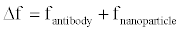(7)

Where, fantibody and fnanoparticle are the characteristic frequency responses of the antibody and nanoparticle, respectively.

Another important consideration is the method of how the conduction of the electrons occurs between the nanoparticle and sensor surfaces. As described by Brust et al.  the conduction is mediated through electron hopping according to the equation below: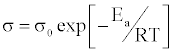(8)

Where σ0 is the conductivity constant, Ea is the activation energy, R is the universal gas constant, and T is the temperature in Kelvin . The activation energy is the energy needed for electron transport to occur. Mathematically, the activation energy is described by the following equation: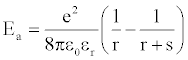(9)

Where e is the charge of an electron, and ε0, εr, r, and s are constants and variables, as previously described.

#### Results

Initial experiments using three particle sizes of 5, 10, and 20 nm resulted in calculated changes to electron transfer resistance as predicted by the models (Figures 4 and 5). Employing the models derived for resistance and capacitance resulted in a dramatic change in frequency of a particular conjugate combination using a simulated range of particle sizes from 0.1 to 20 nm. This relative change in frequency was defined by 1/2πf=R*C, and was plotted as a function of particle size (Figure 5C). A direct correlation (Figure 8) was observed when overlaying the calculated change in frequency with the measured frequency (experimentally) for the three nanoparticle sizes.

Figure 4: (A) Nyquist plots of gold disk electrode with (a) untuned, (b) 20 nm AuNP tuned, (c) 10 nm AuNP tuned, and (d) 5 nm Au NP tuned anti-IL-12 with increasing response (shifted or tuned) by the additional AuNP with a dependency upon size of AuNP. (B) Extrapolated electron transfer resistance (Ret) from the tuned AuNP plotted versus size.

Figure 5: (A) plot of Resistivity (calculated) for activated electron hopping between a nanoparticle and electrode surface (inset schematic showing electron hopping), (B) modeled capacitance versus particle size (inset showing mechanism of electron charging) and (C) calculated frequency effect combining R and C versus particle size. All models were calculated using a spacing of 10 nm.

A review of scientific literature demonstrates the difficulty in finding consistent values for dielectric constants and electrical conductivities of different nanoparticle materials. This is because the dielectric constant for nanoparticles is comprised of both real and imaginary components, and is dependent on the radius, morphology, aspect ratio, and frequency of the nanoparticles . Additionally, the dielectric constant is dependent on the media in which the nanoparticles are present and, in the case of metal nanoparticles, the surface plasmon frequency at a given diameter .

The dielectric constant and electrical conductivity properties of gold, silver, carbon, polystyrene, and silicon quantum dots were researched, and are presented in table 2. These values, as well as our group’s preliminary modeling of gold nanoparticle-antibody conjugates and aforementioned mathematical equations, were used in the development of the mathematical model described herein.

Material Dielectric Constant Electrical Conductivity (Ω-1cm-1) Source
Gold 1.75, 2.63
3-6
10-3-10-9 Link et al. 
Chen et al. 
Silver 3.68-3.95 10-3-10-7 Roy et al. 
Yeh et al. 
Kornain et al 
Carbon 2.4
(graphite)
10-4-10-16 Leonard et al.
Marinho. 
Polystyrene 2.4-3 10-12 Yang et al. 
Barrau et al. 
Silicon QD 11.4 0.1-0.2
(p-type, converted from electrical resistivity values)
Wang et al. 
Snow et al. 
Photopoulos et al. 

Table 2: Summary of the dielectric and electrical conductivity values for different nanoparticle materials.

To determine optimal binding frequency, a concentration gradient of the target must be run (experimentally) first (Figure 6). Nyquist data is gathered for each concentration. For each range of concentrations, the impedance at each frequency and the concentration can be used to calculate a linear (or log) regression in order to obtain slope (responsivity) and tightness of fit (R-square) in order to determine “optimal” binding frequency for detection. This is the frequency in which maximum response is made as well as degree of fit to a linear (or log) calibration curve. Finally the calibration curve can be generated. Then in actual use, a user would simply run the sample (only) and compare impedance measured (at that frequency) with the calibration curve, a correlation factor applied and the resulting data is presented in pg/mL instead of impedance. This data calculation can be applied to untuned and tuned systems.

Figure 6: Experimental results from tuned interleukin-12 (IL-12) showing (A) resulting Nyquist plots of an IL-12 concentration gradient from (a) blank to (i) 10,000 pg/mL of IL-12 binding to 20 nm tuned AuNP. In (B) a tightness of fit from the resulting concentration gradient, calculated for each frequency and plotted reveals that the optimal frequency of binding for this target is at 1 Hz now (tuned from 5 Hz) based upon tightness of fit. (C) Responsivity of tuned system also showing maximal response has also been tuned to 1 Hz over the frequency range by calculation of slope of each concentration bound for each frequency. Finally, optimized correlation plot of impedance at 1 Hz plotted against the concentration gradient for the IL-12: tuned anti-IL-12 system.

A simplistic model can be generated using the equations described herein, and by calculating a frequency change per spacing, three lines can be generated (Figure 7). Plotting onto this model system, one can see the resulting change in frequency from the three experimentally tuned systems for 5, 10 and 20 nm AuNP’s. In this data set, spacing was fixed by simple linker manipulation; the 16-MHDA had a fixed length that creates approximately 5 nm spacing between the sensor and the AuNP attached to the anti-IL-12 antibody. By simple manipulation of linkers, one can affect the spacing readily.

Figure 7: Model of (a) spacing, s=2.5 nm, (b) s=5 nm and (c) s=10 nm of frequency tuning versus particle size. Actual experimental results are also included for (d) 5 nm, (e) 10 nm, and (f) 20 nm AuNP tuned anti-IL-12 sensors.

This proposed design space will allow for specific tuning that uses more parameters than particle diameters (Figure 8). The developed mathematical model shows that the largest detection frequency change or shift can be obtained using a nanoparticle with a small radius and a large electrical conductivity, with frequencies on the order of 100,000 Hz or higher. As the electrical conductivity increases, the detection frequency of the nanoparticle also increases steadily, with asymptotic behavior observed on the surface of the mesh plot corresponding to small radii. The lowest detection frequencies observed on the model presented were just above 10 Hz. As previously mentioned, these high and low detection frequency values were a result of the selected dielectric constant and particle spacing. Adjusting, either the particle spaces or the dielectric constant results in the entire plot shifting upwards or downwards with respect to the frequency axis. Specifically, increasing the particle spacing results in the upward shift of the plot relative to the frequency axis, while decreasing the particle spacing has the opposite effect. Conversely, the opposite trend is observed if the dielectric constant is increased, with a downward shift occurring as the dielectric constant is increased.

Figure 8: Mathematical model showing the design space of the frequency of nanoparticle-antibody conjugates is changed based on different electrical conductivities and sizes of nanoparticles. Changing the dielectric constant value (i.e. changing the material) results in a shift upwards or downwards with respect to the frequency axis. The above plot shows the frequency behavior of 5 nm nanoparticles with a dielectric constant of 6.58, which is the average value of the materials researched. Such a design space can be used when selecting material and nanoparticle sizes in the eventual construction of multiplexed, electrochemical impedance based biosensor.

#### Discussion

It can be inferred from the developed mathematical model that a large range in detection frequencies can be obtained depending on the nanoparticle radius and electrical conductivity. While a large frequency shift is desirable to minimize overlapping of different antibodies on an eventual multiplexed device, careful attention should be made to ensure that nanoparticles for conjugation exhibit appropriate properties for their applications. This is an especially important consideration depending on the morphology of the nanoparticle, media in which the assay is being performed, as well as the dielectric constants of materials are often frequency-dependent [29-31]. This suggests that it may not always be advantageous to have the greatest shift in frequency, but rather a frequency shift that takes the morphology, media and dielectric constant into account. Furthermore, because the spacing of the particles and dielectric constant also affects the detection frequency window, these parameters should be carefully considered as well.

Based on the obtained electrical conductivities for the nanoparticle materials, gold and silver were found to have the highest range of electrical conductivity, and thus corresponded to higher detection frequencies at all specified radius sizes. Both carbon and the silicon quantum dots exhibited intermediary electrical conductivity, with a large detection frequency window at each of the different radii. Polystyrene exhibited the lowest electrical conductivity, and thus corresponded to detection frequencies occupying a lower frequency window than that of gold, silver, carbon, or silicon quantum dots. The findings suggest that certain nanoparticle materials may be more appropriate for tuning than others, depending on the characteristic frequency response of the antibodies being utilized and desired frequency shift.

While the developed mathematical model provides valuable insight into how nanoparticle properties affect the detection frequency, additional parameters have also been taken into considerationthat would increase the accuracy of the model. For example, our model assumes that the nanoparticles have spherical morphologies. More accurate detection frequencies could be obtained by including different nanoparticle morphologies, such as nano prisms, nano rods, and nano plates. However, a major challenge associated with incorporating these morphologies into the model is that the resistance and capacitance calculations become increasingly complex. Furthermore, as evidenced by the conducted literature search, obtaining electrical conductivity, resistivity, and dielectric constants for these morphologies is a challenging task, and varies tremendously depending on the experimental methods utilized. Nonetheless, the mathematical model developed here provides a helpful starting point for assessing the design parameters of nanoparticle-antibody conjugates and allows for identification and potential incorporation of aforementioned complexities.

Convolution of individual frequency signatures of protein biomarkers observed by electrochemical impedance spectroscopy poses a challenge for simultaneous detection of such molecules for disease detection or profiling. Frequency tuning method should promise as a novel solution for utilization of these unique disease signatures. Here, we present a mathematical model and analysis of experimental data to further the tuning design space previously demonstrated by our group and experimentally pushed those boundaries. Gold nanoparticle size changes of 5, 10, and 20 nm demonstrated a 10.0, 4.8, and 1.0 Hz shift in frequency, respectively. This shift will allow for marker-antibody pairs whose frequency of optimal binding may overlap to be tuned away from one another for future multiplexed simultaneous detection. However, use of this model reveals that dramatic changes are possible by switching material and/or spacing, as well as nanoparticle size. Future work will include investigation of nanoparticle compositions (pure and/or mixed), mixed markers, and development of instrumentation for simultaneous detection of mixed markers via a single, disposable electrode. Additionally, time based impedance will be performed to begin the transition to a more applicable device. Once single frequency impedance signals can be generated and used to perturb single biomarker-antibody pairs, mixed frequency or multitonal signals can be used to observe co-immobilized mixed markers-antibody binding, simultaneously.

#### Acknowledgements

This work was supported in part by the Biodesign Institute at Arizona State University and the Fulton Undergraduate Research Initiative.

#### References

Select your language of interest to view the total content in your interested language

### Article Usage

• Total views: 12665
• [From(publication date):
specialissue-2013 - Sep 19, 2019]
• Breakdown by view type
• HTML page views : 8785

## Post your commentCan't read the image? click here to refresh
###### Peer Reviewed Journals

Make the best use of Scientific Research and information from our 700 + peer reviewed, Open Access Journals

International Conferences 2019-20

Meet Inspiring Speakers and Experts at our 3000+ Global Annual Meetings

Top